PHY_10_64_V1_DM_Faraday law of induction
Оценка 4.6

# PHY_10_64_V1_DM_Faraday law of induction

Оценка 4.6
docx
08.05.2020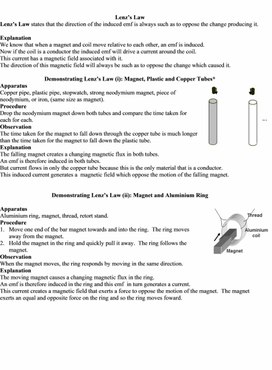PHY_10_64_V1_DM_Faraday law of induction.docx

Lenz’s Law

Lenz’s Law states that the direction of the induced emf is always such as to oppose the change producing it.

Explanation

We know that when a magnet and coil move relative to each other, an emf is induced.

Now if the coil is a conductor the induced emf will drive a current around the coil.

This current has a magnetic field associated with it.

The direction of this magnetic field will always be such as to oppose the change which caused it.

Demonstrating Lenz’s Law (i): Magnet, Plastic and Copper Tubes*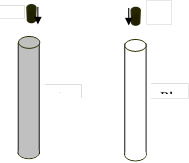Apparatus

Copper pipe, plastic pipe, stopwatch, strong neodymium magnet, piece of neodymium, or iron, (same size as magnet).

Procedure

Drop the neodymium magnet down both tubes and compare the time taken for each for each.

Observation

The time taken for the magnet to fall down through the copper tube is much longer than the time taken for the magnet to fall down the plastic tube.

Explanation

The falling magnet creates a changing magnetic flux in both tubes.
An emf is therefore induced in both tubes.
But current flows in only the copper tube because this is the only material that is a conductor.

This induced current generates a  magnetic field which oppose the motion of the falling magnet.

Demonstrating Lenz’s Law (ii): Magnet and Aluminium Ring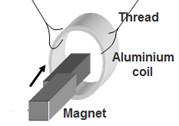Apparatus

Aluminium ring, magnet, thread, retort stand.

Procedure

1.      Move one end of the bar magnet towards and into the ring.  The ring moves away from the magnet.

2.      Hold the magnet in the ring and quickly pull it away.  The ring follows the magnet.

Observation

When the magnet moves, the ring responds by moving in the same direction.

Explanation

The moving magnet causes a changing magnetic flux in the ring.
An emf is therefore induced in the ring and this emf  in turn generates a current.

This current creates a magnetic field that exerts a force to oppose the motion of the magnet.  The magnet exerts an equal and opposite force on the ring and so the ring moves foward.

Questions

1.      Explain the term emf

2.      What is electromagnetic induction?

3.       A magnet and a coil can be used to produce electricity.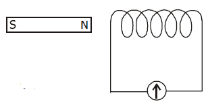How would you demonstrate this?

4.

(i)     A coil of wire is connected to a sensitive galvanometer as shown in the diagram.

What is observed when the magnet is moved towards the coil?

(ii)  Explain why this occurs.

(iii)            Describe what happens when the speed of the magnet is increased.

5.

(i)     Draw a sketch of the apparatus Michael Faraday used to generate electricity.

(ii)  What name is given to the generation of electricity discovered by Michael Faraday?

(iii)            What energy conversions takes place in Faraday’s experiment

(iv) How does Faraday’s experiment show that a changing magnetic field is required to generate electricity?

6.      State Faraday’s law of electromagnetic induction.

7.      State Lenz’s law of electromagnetic induction.

8.

State the laws of electromagnetic induction.

9.      Describe an experiment to demonstrate electromagnetic induction.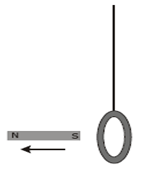10.  Describe an experiment to demonstrate Faraday’s law.

Lenz Law

11.

(i)     A light aluminium ring is suspended from a long thread as shown in the diagram.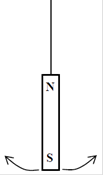When a strong magnet is moved away from it, the ring follows the magnet.

Explain why.

(ii)  What would happen if the magnet were moved towards the ring?

12.

(i)     A bar magnet is attached to a string and allowed to swing as shown in the diagram.
A copper sheet is then placed underneath the magnet. Explain why the amplitude of the swings decreases rapidly.

(ii)  What is the main energy conversion that takes place as the magnet slows down?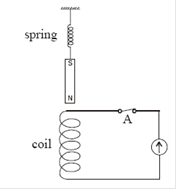13.

(i)     A small magnet is attached to a spring as shown in the diagram.
The magnet is set oscillating up and down. Describe the current flowing in the circuit.

(ii)  If the switch at A is open, the magnet will take longer to come to rest. Explain why.

Magnetic flux and Faraday’s Law

14.

Define magnetic flux.

15.

A coil has 5000 turns.

What is the emf induced in the coil when the magnetic flux cutting the coil changes by 8 × 10–4 Wb in 0.1 s?

16.

What is the average emf induced in a coil of 20 turns when the magnetic flux cutting it decreases from 2.3 Wb to 1.4 Wb in 0.4 s?

17.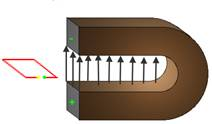(i)     A metal loop of wire in the shape of a square of side 5 cm enters a magnetic field of flux density 8 T.

The loop is perpendicular to the field and is travelling at a speed of 5 m s–1.

How long does it take the loop to completely enter the field?

(ii)  What is the magnetic flux cutting the loop when it is completely in the magnetic field?

(iii)            What is the average emf induced in the loop as it enters the magnetic field?

{the diagram wasn’t in the original question but it may be useful here to help picture the situation}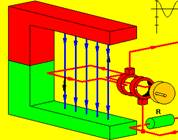18.

(i)     A square coil of side 5 cm lies perpendicular to a magnetic field of flux density 4.0 T.

The coil consists of 200 turns of wire. What is the magnetic flux cutting the coil?

(ii)  The coil is rotated through an angle of 90o in 0.2 seconds. Calculate the magnitude of the average e.m.f. induced in the coil while it is being rotated.

{the diagram wasn’t in the original question but it may be useful here to help picture the situation}

19.

Read the following passage and answer the accompanying questions.

The growth of rock music in the 1960s was accompanied by a switch from acoustic guitars to electric guitars. The operation of each of these guitars is radically different.

The frequency of oscillation of the strings in both guitars can be adjusted by changing their tension. In the acoustic guitar the sound depends on the resonance produced in the hollow body of the instrument by the vibrations of the string. The electric guitar is a solid instrument and resonance does not occur.

Small bar magnets are placed under the steel strings of an electric guitar, as shown. Each magnet is placed inside a coil and it magnetises the steel guitar string immediately above it. When the string vibrates the magnetic flux cutting the coil changes, an emf is induced causing a varying current to flow in the coil. The signal is amplified and sent to a set of speakers.

Jimi Hendrix refined the electric guitar as an electronic instrument. He showed that further control over the music could be achieved by having coils of different turns.

(Adapted from Europhysics News

(a)   Why must the strings in the electric guitar be made of steel?

(b)   Why does the current produced in a coil of the electric guitar vary?

(c)   What is the effect on the sound produced when the number of turns in a coil is increased?

20.  The peak voltage of the mains electricity is 325 V. Calculate the rms voltage of the mains.

21.  Sketch a voltage-time graph of the 230 V supply.

22.  Electricity produced in a generating station is a.c. What is meant by a.c.?

23.

Sketch a graph to show the relationship between current and time for

(i)                 alternating current

(ii)              direct current.

24.  What is a diode?

25.  Give an example of a device that contains a rectifier.

26.  Name a device that is based on electromagnetic induction.

Transformers

27.  What is a transformer used for?

28.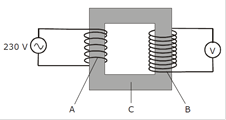The transformer is a device based on the principle of electromagnetic induction.

Name two devices that use transformers.

29.  Name the parts of the transformer labelled A, B and C in the diagram.

30.  How is the iron core in a transformer designed to make the transformer more efficient?

31.  The efficiency of a transformer is 90%. What does this mean?

32.  State one energy conversion that takes place in an electrical generator.

33.  The mains electricity supply (230 V) is connected to the input coil of a transformer which has 400 turns. The output coil has 100 turns. What is the reading on the voltmeter?

34.  The input coil of a transformer has 400 turns of wire and is connected to a 230 V a.c. supply while the output coil as 1200 turns. What is the voltage across the output coil?

35.  The input voltage is 230 V. The input coil has 4600 turns and the output coil has 120 turns.

Calculate the output voltage.

Self-Induction

36.

(i)     A resistor is connected in series with an ammeter and an ac power supply. A current flows in the circuit. The resistor is then replaced with a coil. The resistance of the circuit does not change.

What is the effect on the current flowing in the circuit?

(ii)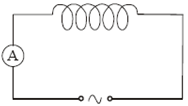Justify your answer

37.

(i)     In an experiment, a coil was connected in series with an ammeter and an a.c. power supply as shown in the diagram. Explain why the current was reduced when an iron core was inserted in the coil.

(ii)  Give an application of the principle shown by this experiment.

Скачано с www.znanio.ru

## Lenz’s Law Lenz’s Law states that the direction of the induced emf is always such as to oppose the change producing it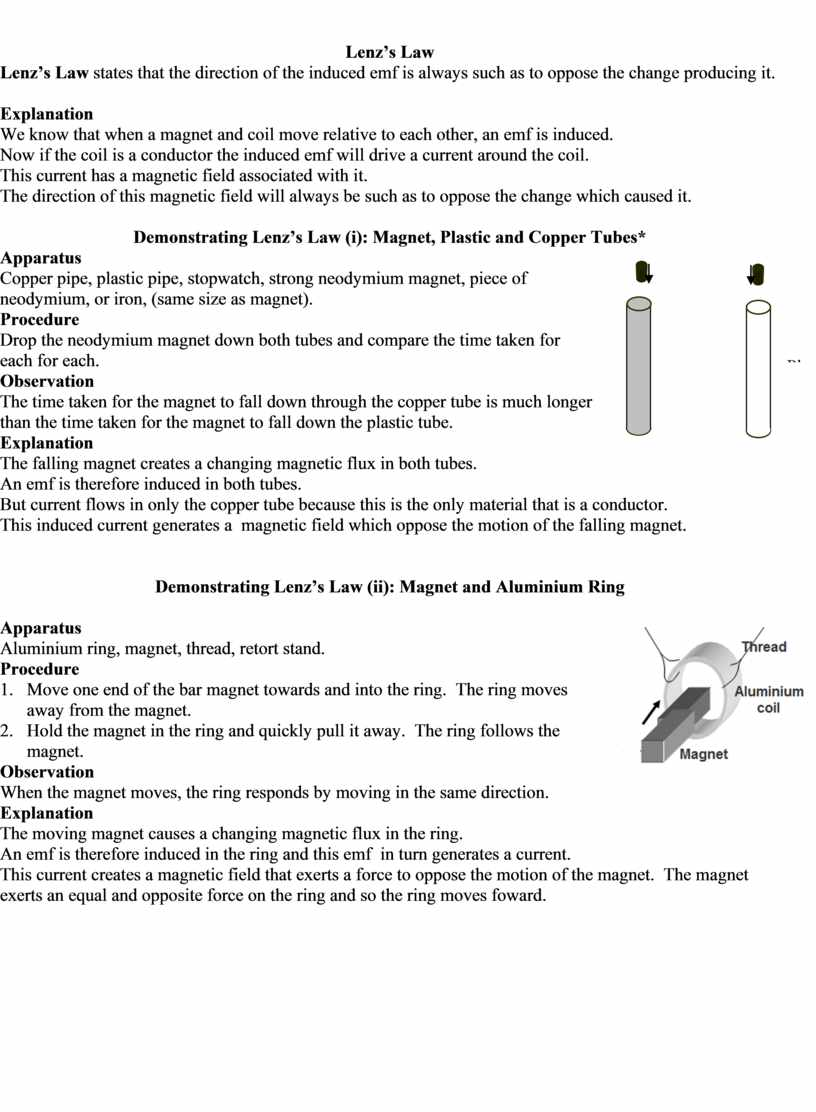## A small magnet is attached to a spring as shown in the diagram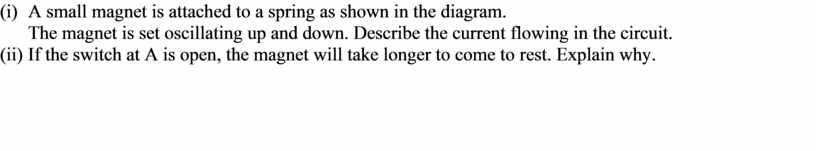Скачать файл